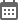CMAT Percentile Predictor - 2022

Predict your CMAT percentile to estimate your performance compared to other test takers appearing for the CMAT exam. Percentile estimates are based on historical data in past examsCMAT Percentile Predictor 2022

CMAT Percentile Predictor 2022: The Common Management Admission Test (CMAT) percentile predictor enables you to check your CMAT 2022 percentile right after the conclusion of the CMAT entrance test. CMAT percentile predictor uses an advanced algorithm to assess your CMAT percentile. The CMAT 2022 percentile predictor is a tool devised after considering a few factors such as CMAT exam overall and sectional difficulty level, number of test-takers, and among other factors. In order to know CMAT 2022 percentile, candidates can use Careers360 CMAT percentile predictor tool.

How to use CMAT Percentile Predictor 2022?

The usage of the CMAT percentile predictor 2022 tool is very easy. All you need to do is enter your estimated CMAT 2022 score out of 400, sectional marks, and CMAT registration number (optional) and this tool will fetch your CMAT 2021 percentile. Check out the step by step guide to use the CMAT percentile predictor tool and get your CMAT 2022 percentile.

Step 1- Enter the following details.

• Score out of 400
• QTDI marks
• LR marks
• LC marks
• Enter CMAT registration number (optional)
• Enter date of birth in mm/dd/yyyy format (optional)

Step 2- Now, click on the “Predict My percentile” button

Step 3- On submission of the details, the CMAT percentile predictor tool will use its algorithm to calculate the probable CMAT percentile and display the same

How helpful is the CMAT Percentile Predictor?

The benefit of using the CMAT percentile predictor is to give the candidates an idea about their expected percentile in CMAT exam.The CMAT 2022 percentile predictor will calculate the sectional & overall percentiles as per the scores filled in by the test takers and based on this calculation, it will display CMAT result 2022 that can be secured by the candidates.

CMAT 2022 Percentile Predictor: How is the CMAT percentile calculated?

CMAT percentile is calculated on the basis of candidate’s percentile vs number of students appearing in CMAT 2022 entrance test. For this, NTA applies the below formula.

Percentile P = 100 x Number of candidates appeared in the examination with raw marks equal to or less than the candidate/Total number of candidates appeared

For example, if the number of appeared candidates in CMAT entrance test is 1000 and the highest scored marks (350/400) then he/she percentile will be 1 and CMAT percentile will be 100 x 9999/10,000= 99.99 CMAT percentile.

CMAT Result 2022
The National Testing Agency (NTA) will release the CMAT 2022 result. Candidates will be able to check their CMAT 2022 result in online mode on the official website of NTA. For checking the CMAT results, candidates will have to use their login credentials such as CMAT application number and date of birth.

Other Rank Predictors